Quadratic Equations: Factoring and Square Rooting MathBitsNotebook.com Terms of Use   Contact Person: Donna Roberts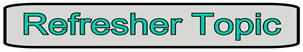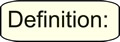A quadratic equation is a polynomial equation of degree two, which can be written in the form ax2 + bx + c = 0, where x is a variable and a, b and c are constants with a ≠ 0.Solving quadratic equations:
Solving a quadratic equation can range from being a simple task, to being a challenge. These refresher pages build an arsenal of strategies for solving quadratic equations.
 • solve by factoring (on this page) • solve by square root method (on this page) • solve by completing the square • solve by using the quadratic formula • solve by graphingTo solve a quadratic equation by factoring:
For more details on the process of factoring, see the Algebra 1 Section on Factoring. The information presented on this page is meant as a refresher of those factoring skills, as they pertain to quadratic equations.

 Factoring Method 1. Express the equation in the form ax2 + bx + c = 0. 2. Factor the left hand side (if 0 is on the right). 3. Set each of the two factors equal to zero. 4. Solve for x to determine the roots (or zeros).

Simple quadratic equations with rational roots can be solved by factoring. Let's refresh our memories on factoring these simple quadratic equations as they appear in different situations.
See Algebra 1 Factoring for more examples.

Examples of Solving Quadratic Equations by Factoring:
 Factoring with GCF (greatest common factor): Solve:  4x2 - 28x = 0 4x(x - 7) = 0 4x = 0;    x - 7 = 0 x = 0;       x = 7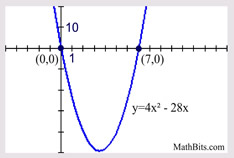Find the largest value which can be factored from each term on the left side of the quadratic equation. The roots (zeros) correspond to the locations of the x-intercepts of the function y = 4x2 - 28x.

 Factoring Trinomial with Leading Coefficient of One: Solve:  x2 + 2x - 15 = 0 (x + 5)(x - 3) = 0 x + 5 = 0;    x - 3 = 0 x = -5;       x = 3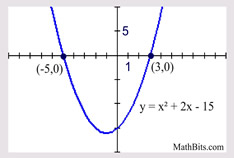When the leading coefficient is one, the product of the roots will be the constant term, and the sum of the roots will be the coefficient of the middle x-term.

 Factoring Difference of Two Squares: Solve:  x2 - 81 = 0 (x + 9)(x - 9) = 0 x + 9 = 0;    x - 9 = 0 x = -9;       x = 9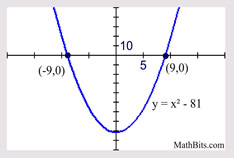Remember the pattern for the difference of two squares, where the factors are identical except for the sign between the terms.

 Factoring Trinomial with Leading Coefficient Not One: Solve:  3x2 + 11x - 4 = 0 (3x - 1)(x + 4) = 0 3x - 1 = 0;    x + 4 = 0 x = 1/3;       x = -4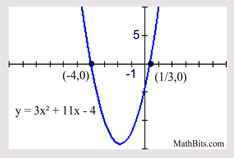Life gets more difficult when the leading coefficient is not one. Check out the Factoring section to see more strategies for factoring these pesky problems.

 Where's the x2 ? Solve:  2x(x + 4) = x - 3 2x2 + 8x = x - 3 2x2 + 7x + 3 = 0 (2x + 1)(x + 3) = 0 2x + 1 = 0;    x + 3 = 0 x = - 1/2;       x = -3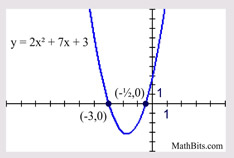Sometimes you have to "work" on the equation to get the needed quadratic form. In this case, distribute, and the x2 will appear.

 Dealing with Proportions: Solve: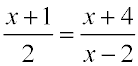2(x + 4) = (x + 1)(x - 2) 2x + 8 = x2 - x - 2 0 = x2 - 3x - 10 0 = (x - 5)(x + 2) x - 5 = 0;    x + 2 = 0 x = 5;       x = -2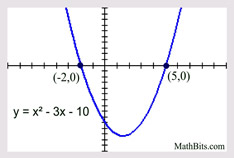x2 may appear when cross multiplying ("product of the means equals product of the extremes") is employed in a proportion.To solve a quadratic equation by taking a square root:

 Square Root Method (ax2 is the only variable term) 1. Isolate the ax2 term on one side of the equation. 2. Take the square root of both sides. 3. Remember to use ±, as there are two solutions. 4. Express the roots (or zeros).

The square root method applies only to one specific situation. This method works when there is no middle bx-term in the equation. The only variable in the equation is an x2-term.

Examples of Solving Quadratic Equations by Square Root Method:
 Easiest Set-Up (has "x2 = __"): Solve:    x2 = 121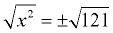x = ±11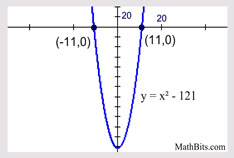We saw this example under the previous method of factoring. Here it is again, being solved by the square root method. Notice the answers are the same.

 Difference of Two Squares: Solve:    x2 - 81 = 0 x2 = 81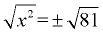x = ± 9We saw this example under the previous method of factoring. Here it is again, being solved by the square root method. Notice the answers are the same.

 Only variable is the x2 term, with subtraction of constant. Solve:    x2 - 12 = 0 x2 = 12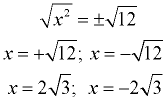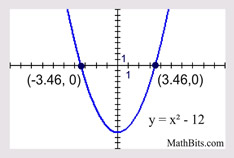This example shows the numeric value (the constant), subtracted from the x2 term. Approximate decimal answers: x = -3.464102 and x = 3.4641016

 Only variable is the x2 term, with addition of constant. Solve:    x2 + 9 = 0 x2 = -9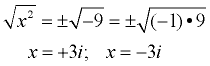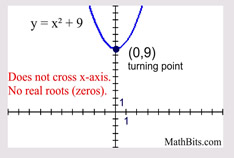This example shows the numeric value (the constant),  added to the x2 term, which creates "complex" answers containing the imaginary "i".

There are tools more powerful than factoring and square root method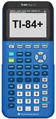For calculator help with graphing parabolas click here.